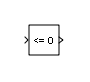# Compare To Zero

Determine how signal compares to zero

• Library:
• Simulink / Logic and Bit Operations

HDL Coder / Logic and Bit Operations

•## Description

The Compare To Zero block compares an input signal to zero. Specify how the input is compared to zero with the Operator parameter.

The output is `0` if the comparison is false, and `1` if it is true.

## Ports

### Input

expand all

Input signal, specified as scalar, is compared with zero. If the input data type cannot represent zero, parameter overflow occurs. To detect this overflow, go to the Diagnostics > Data Validity pane of the Configuration Parameters dialog box and set Parameters > Detect overflow to `warning` or `error`.

In this case, the block compares the input signal to the ground value of the input data type. For example, if you have an input signal of type `fixdt(0,8,2^0,10)`, the input data type can represent unsigned 8-bit integers from 10 to 265 due to a bias of 10. The ground value is 10, instead of 0.

Data Types: `half` | `single` | `double` | `int8` | `int16` | `int32` | `int64` | `uint8` | `uint16` | `uint32` | `uint64` | `Boolean` | `fixed point`

### Output

expand all

The output is `0` if the comparison is false, and `1` if it is true.

The block output is `uint8` or `boolean`, depending on your selection for the Output data type parameter.

Data Types: `uint8` | `Boolean`

## Parameters

expand all

This parameter can have the following values:

• `==` — Determine whether the input is equal to zero.

• ~`=` — Determine whether the input is not equal to zero.

• `<` — Determine whether the input is less than zero.

• `<=` — Determine whether the input is less than or equal to zero.

• `>` — Determine whether the input is greater than zero.

• `>=` — Determine whether the input is greater than or equal to zero.

#### Programmatic Use

 Block Parameter: `relop` Type: character vector Values: `'=='` | `'~='`| `'<'` |`'<='`| `'>='`| `'>'` Default: `'<='`

Specify the data type of the output, `boolean` or `uint8`.

#### Programmatic Use

 Block Parameter: `OutDataTypeStr` Type: character vector Values:`'boolean'` | `'uint8'` Default: `'boolean'`

#### Programmatic Use

 Block Parameter: `ZeroCross` Type: character vector Values: `'off'` | `'on'` Default: `'on'`

## Block Characteristics

 Data Types `Boolean` | `double` | `fixed point` | `half` | `integer` | `single` Direct Feedthrough `yes` Multidimensional Signals `yes` Variable-Size Signals `yes` Zero-Crossing Detection `yes`

## Version History

Introduced before R2006a